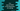# C++ program to find the sum of ASCII values of all characters of a string## C++ program to find the sum of ASCII values of all characters of a string:

In this post, we will learn how to find the sum of ASCII values of all characters of a string. We will read one string as the input from the user and print the sum of ASCII of all characters in the string.

We will also show the ASCII values of each character of the string.

We can use a loop to iterate over the characters of the string and find out the sum.

## Example 1: Find the sum of ASCII of all characters of a string by using a for loop:

Let’s try this by using a for loop. Below is the complete program:

``````#include <iostream>
using namespace std;

int main()
{
int sum = 0;
char str;

cout << "Enter a string: " << endl;
cin.getline(str, 50);

for (int i = 0; str[i] != '\0'; i++)
{
cout << "ASCII of " << str[i] << " is " << (int)str[i] << endl;
sum += (int)str[i];
}

cout << "Total sum: " << sum << endl;
}``````
• sum is an integer value and it is initialized as 0. This variable is to hold the sum of all ASCII values of the string characters.
• str is a character array to hold the user input string.
• It asks the user to enter a string and reads that string by using cin.getline. It puts that in the str character.
• It uses a for loop to iterate through the characters of the string one by one. It runs until the character is ‘\0’.
• Inside the loop, it prints the ASCII value of the character it is iterating. It type casts the character to integer and adds that value to the sum variable.
• After the for loop ends, it prints the value of the sum, i.e. the sum of all ASCII values of the characters.

If you run this program, it will print output as like below:

``````Enter a string:
world
ASCII of w is 119
ASCII of o is 111
ASCII of r is 114
ASCII of l is 108
ASCII of d is 100
Total sum: 552

Enter a string:
hello world !!
ASCII of h is 104
ASCII of e is 101
ASCII of l is 108
ASCII of l is 108
ASCII of o is 111
ASCII of   is 32
ASCII of w is 119
ASCII of o is 111
ASCII of r is 114
ASCII of l is 108
ASCII of d is 100
ASCII of   is 32
ASCII of ! is 33
ASCII of ! is 33
Total sum: 1214``````

## Method 2: Find the sum of ASCII values of all characters in a string using a while loop in C++:

We can also use a while loop to do the same thing. The above program can be written by using a while loop. The while loop will run until the current iterating character is \0. It will keep running and find out the sum of all ASCII values of the characters in that string.

Below is the complete program:

``````#include <iostream>
using namespace std;

int main()
{
int sum = 0, i = 0;
char str;

cout << "Enter a string: " << endl;
cin.getline(str, 50);

while (str[i] != '\0')
{
cout << "ASCII of " << str[i] << " is " << (int)str[i] << endl;
sum += (int)str[i];
i++;
}

cout << "Total sum: " << sum << endl;
}``````

It is similar to the above program and it will give one similar result.

The only differences are we have initialized i before the loop starts and the increment of i is done at the end of the loop.